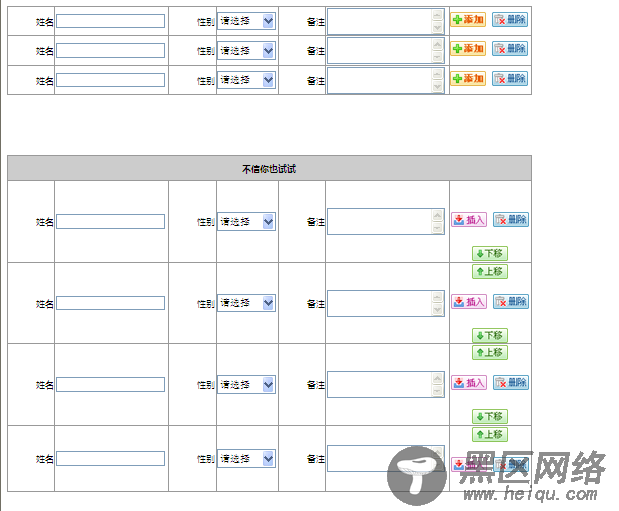# 自己动手制作jquery插件之自动添加删除行功能介Boolean类型的参数，如果你要完全的复制某个dom对象，包括它上面的事件的话，就可以用clone(true)了，这样也少了再次绑定事件的困绕，所以这个插件的回调方法，其实很难用到。添加行的代码如下：

function addRow(num, isInsert, target) {
for (var i = 0; i < num - count; i++) {
var temp = content.find("." + settings.tempRowClass).first().clone(true);
temp.find("input").val("");
temp.find("select").val("-1");
temp.find("textarea").val("");
temp.find(":checkbox").attr("checked", false);
temp.attr("id", "");
temp.find("input,tr,textarea,select,:checkbox,tbody").attr("id", "");
if (temp.find(".richText").length > 0) {
temp.find(".ke-container").remove();
}
temp.find(".richText,input").each(function() {
\$(this).attr("id", "txt_" + Math.round(Math.random() * new Date().getTime()))
\$(this).show();
});
if (isInsert) {
temp.insertBefore(target.closest("." + settings.tempRowClass));
} else {
content.append(temp);
}
}
if (num < count) {
for (var j = count - 1; j >= num; j--) {
delRow(content.find("."+settings.tempRowClass).eq(j));
}
} else {
}
count = content.find("." + settings.tempRowClass).length;
settings.changeInput.val(count);
sumIndex();
showhideBtn();
};